• 根号n段归并排序算法时间复杂度分析过程： 1.合并 根号n向下取整 段子数组使用的是自底向上两两归并的策略 2.根号n段归并排序算法时间复杂度的数学推导
• 广度优先搜索构建迷宫（BFS算法）动态构建过程的python 源代码，详情请移步本人博客<迷宫与寻路可视化（二）广度优先搜索构建迷宫（BFS算法）>
• 选择排序、冒泡排序、归并排序、快速排序、插入排序的算法原理。不同排序算法时间效率的经验分析方法，验证理论分析与经验分析的一致性。java
• 算法复杂度分为时间复杂度和空间复杂度。 其作用： 时间复杂度是指执行算法所需要的计算工作量； 而空间复杂度是指执行这个算法所需要的内存空间。 （算法的复杂性体现在运行该算法时的计算机所需资源的多少上，...
• 应用马尔科夫链模型证明了遗传禁忌搜索算法是以概率1收敛到全局最优解的,并应用求解随机算法时间复杂度的方法,即求解算法的期望收敛时间,估算了该算法的时间复杂度,结果证明该算法的时间复杂度与所得解的多样性、...
• 具体点来讲就是我们在实现某一种算法的时候，最终目的就是要求计算机(CPU)在最短的时间内，用最少的内存稳定的输出正确的结果。这一章节主要来理解 “快”，至于“省” 和 “稳”，我会在后续章节进行讲解。 那如何...
金庸武侠中描述一种武功招式的时候，经常会用到 “快、准、狠” 这3个字眼。同样，在计算机中我们衡量一种算法的执行效率的时候也会考量3个方面：“快、省、稳”。
具体点来讲就是我们在实现某一种算法的时候，最终目的就是要求计算机(CPU)在最短的时间内，用最少的内存稳定的输出正确的结果。这一章节主要来理解 “快”，至于“省” 和 “稳”，我会在后续章节进行讲解。
那如何来判断某一段代码运行的是否足够快呢？？有没有一种标准让我们能迅速判断出某A算法比某B算法好呢？？大O复杂度表示法 就是我们要寻找的答案，一般情况下，大O复杂度表示法是用来表示算法性能最常见的正式标记法，接下来就一起来看下这个大O复杂度表示法是个什么东东。
算法时间复杂度的由来
在理解什么是时间复杂度之前，我们需要先了解为什么需要复杂度分析。为了更形象的理解这个问题，我们用一段具体的代码来深入分析。这里有段非常简单的代码，实现了 1,2,3…n 的累加和。
案例1：
// 计算 1, 2, 3…n 的累加和
public class Test {
int cal(int n) {
int sum = 0;
int i = 1;
for (; i <= n; ++i) {
sum = sum + i;
}
return sum;
}
}

如果我们要测试以上面这段代码的执行效率，该如何去测试呢 ？？
起初，我们能想到最简单最直接的方法就是把代码在机器上跑一遍，通过统计、监控，就能得到这段代码所执行的时间和占用的内存大小。既然是这样那为什么还要做时间、空间复杂度分析呢？复杂度分析会比我实实在在跑一遍得到的数据更准确吗？
首先，我可以肯定的说，这种评估算法的执行效率是正确的，并且在某些数据结构和算法的书籍中还专门给这种方法起了个名字—事后统计法。但是这种统计方法有非常大的局限性
1 测试结果极度依赖测试环境 测试环境中的硬件不同会对测试结果有很大的影响。比如案例1的代码分别跑在Intel Core i9 和 Intel Core i3的CPU上运行，很明显i9要比i3快很多。再比如我们在同一时刻在i9上执行运算1024/3，并在i3上运算10+10。正常来讲 10+10 操作比 1024/3 的操作简单很多，但是因为硬件性能的影响，导致i9处理器更快的得出结果。难道我们能说 1024/3 比 10+10 算法性能更高？显然不能！即使是一个初级工程师也应该知道除法运算比加法运算消耗更高！这样就造成我们很难用一个统一的标准去衡量执行时间。
2 测试结果受数据规模的影响很大 后续我们会讲到多种排序算法。对于同一种排序算法，比如快速排序或者插入排序，待排序数据的有序度不一样，排序的执行时间就会有很大差别。另外，对于不同的排序算法，测试数据规模不同也可能导致无法真实的反应排序的性能。比如，我们手上有一组小规模的数据需要做排序操作 int arr[] = {4, 10, 42, 1, 9}; 如果分别使用插入排序和快速排序对 arr 进行排序，我们会发现插入排序比快速排序更快。但是这样不能说明插入排序的性能就比快速排序更高。因为随着arr规模的增长，它的性能会越来越低于快速排序。
综上所述，我们需要一个不用具体的测试数据来测试，用“肉眼”就可以粗略的估计算法的执行效率的方法。而这种方法就是我们今天要讲的 时间复杂度分析方法。

代码复杂度的分析过程
算法的执行时间等于它所有基本操作执行时间之和， 而一条基本操作的执行时间等于它执行的次数和每一次执行的时间的积，如下：
算法的执行时间 = 操作1 + 操作2 + ... + 操作n
操作的执行时间 = 操作执行次数 * 执行一次的时间

我们还是以案例1的代码为例。我们知道java代码经过编译之后，最终会被以字节码的方式由JVM来解释执行相应的指令，那我们可以通过如下命令，分别对Test.java进行编译并查看字节码
javac Test.java    // 编译java代码，生成.class字节码文件
javap -c Test      // 使用javap工具查看字节码指令

执行上述的javap命令之后，得到的字节码指令如下: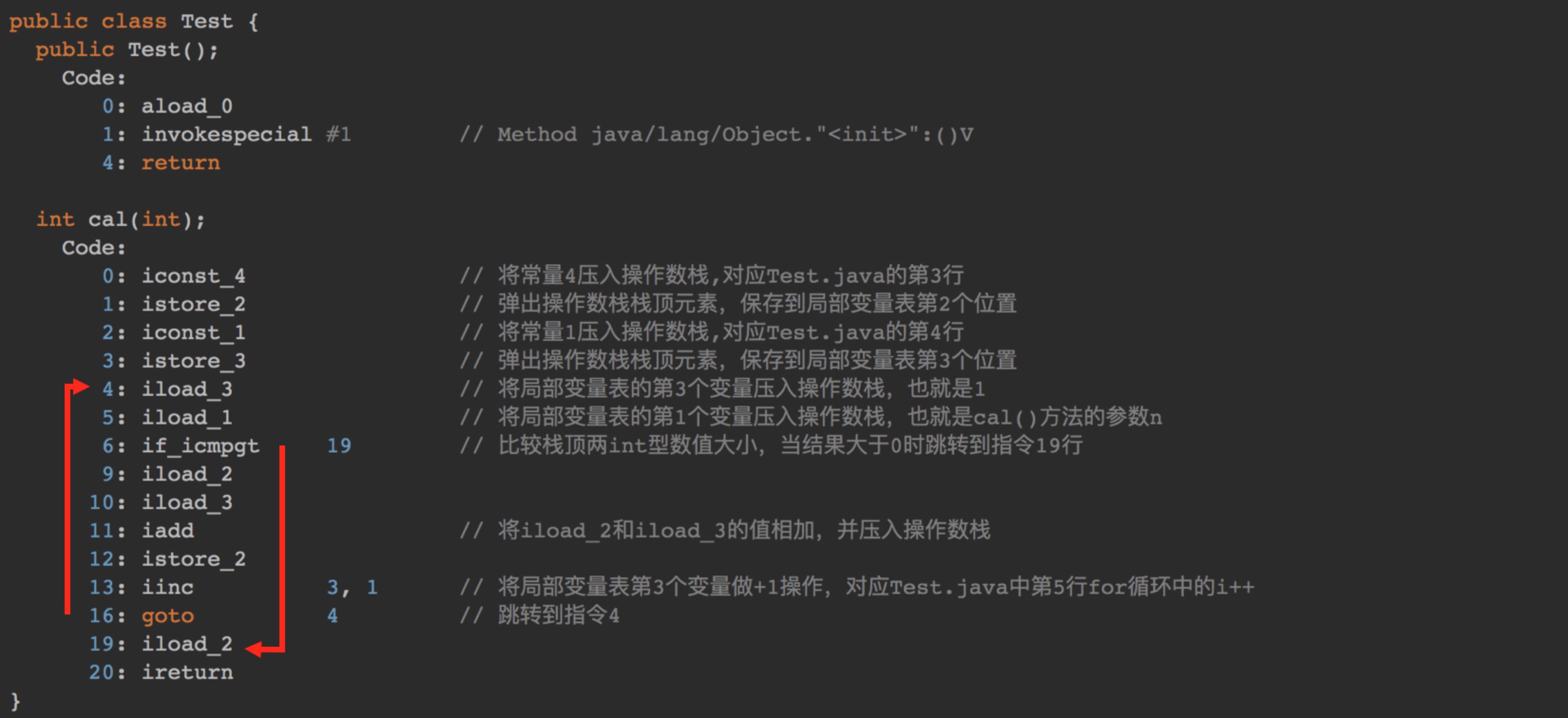可以看出，案例1主要包含的字节码指令就是 iconst_ istore_ iload_ if_icmpgt iadd 等指令，具体每一条指令所代表的意义我已经添加注释(这块内容有点多,但建议耐心仔细阅研读一下，彻底理解每一条指令的意义，也有助于你理解Java代码的运行机制)
然而存在一个问题，不同的编程语言，不同的编译器，或不同的CPU等因素将导致执行一次指令操作的时间各不相同，这样的结果会使算法的比较产生歧义， 于是我们假定所有计算机执行相同的一次指令操作所需时间相同，统一定为 unit_time 。而把算法中基本操作所执行的 执行次数n 作为量度。就是说我们把算法的 运行时间 简单地用基本操作的 运行次数 来代替了, 进而将分析 运行时间 转移到某一行代码的 运行次数

其中unit_time在不同的CPU上可能不一样，比如在i9 Core上有可能是0.01ms，而在i3 Core上可能就是0.05ms。在这个假设的基础上，我们就可以计算出这段代码的总执行时间。

字节码指令是自上而下顺序执行的，所以从指令0开始执行。0-3指令都执行一遍，也就是 **unit_time * 4** 。从指令 4 到指令 19，从图中两个红色箭头也能看出存在循环操作，而
循环的依据就是if_icmpgt指令。
if_icmpgt 指令判断的是操作数栈顶的两个元素的大小，也就是 i 与 n 的大小，因为从指令 4 到指令 19 一共包含10条指令，所以4~19的指令执行次数为：10 * n * unit_time。
综上所述，这段代码总的执行时间就是：

4 * unit_time + 10 * n * unit_time = (10n + 4) * unit_time

按照这个分析思路，我们再来看下面这段代码。
案例2
void cal(int n) {
int sum = 0;
int i = 1;
int j = 1;
for (; i <= n; ++i) {
j = 1;
for (; j <= n; ++j) {
sum = sum +  i * j;
}
}
}

案例2的代码与案例1的唯一区别就是多了一层for循环。经过javac和javap之后的字节码指令如下：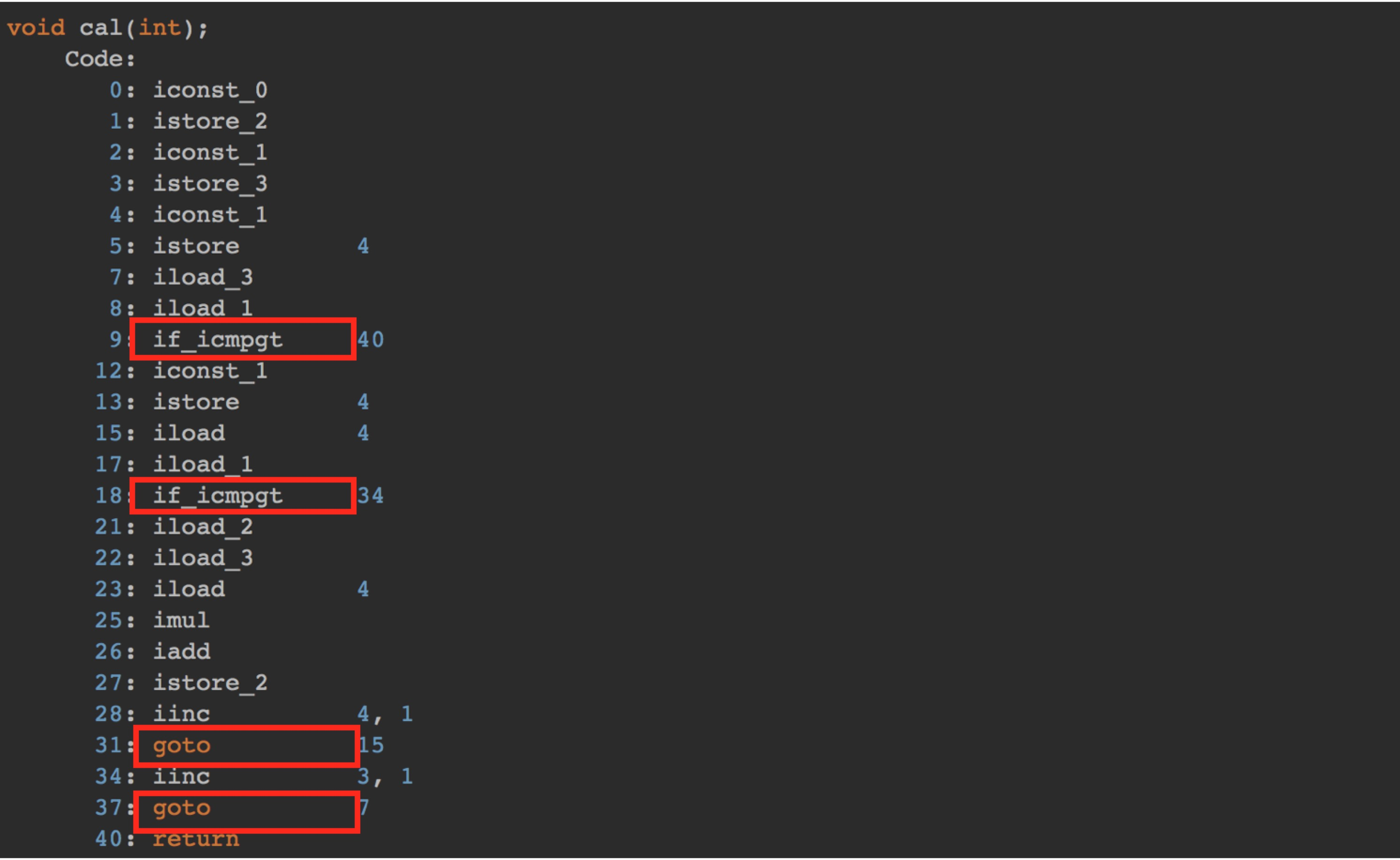分析过程同案例1的一样，具体就不再赘述了。最终分析案例2总的执行时间为：(45n² + 5n + 5) * unit_time
算法时间复杂度表示法
上面我们通过分析字节码指令，计算出案例1和案例2代码片段的具体运行时间，如下：

案例1运行时间 -> (10n + 4) * unit_time案例2运行时间 -> (45n² + 5n + 5) * unit_time

尽管我们不知道 unit_time 的具体值，但是通过对案例1和案例2代码执行时间的推导过程，我们可以得到一个非常重要的规律，那就是 所有代码的执行时间 T(n) 与每行代码的执行次数 n 成正比。
我们可以把这个规律总结成一个公式，注意，大O要登场了
 T(n) = O(f()n)

来解释一下这个公式：T(n)表示代码的执行时间；n表示数据规模的大小；f(n)是一个函数，表示每行代码执行的次数总和。函数f(n)外部的大O表示代码的执行时间 T(n) 与 f(n) 表达式成正比。

注意：大 O 时间复杂度实际上并不具体表示代码真正的执行时间，而是表示代码执行时间随数据规模增长的变化趋势，所以，也叫作渐进时间复杂度(aymptotic time complexity)，简称时间复杂度。或者说它表达的是随着数据规模n的增长，代码执行时间的增长趋势(类似于物理中的加速度)

既然大O表示的是一种增长趋势而不是具体时间，那在我们先前计算出的等式中的 unit_time 也就没什么太大意义了。所以，我们可以将等式中的 unit_time 去掉。

案例1运行时间 -> (10n + 4) *unit_time案例2运行时间 -> (45n² + 5n + 5) *unit_time

最终案例1中的 T(n) = O(10n + 4)，案例2中的 T(n) = O(45n² + 5n + 5)。这就是大 O 时间复杂度表示法。
现在我们了解了大O表示法的演进过程，接下来我们再把视线重新放到案例2的复杂度表示上 T(n) = O(45n² + 5n + 5)，细看之下，此表达式的函数f(n)部分由3部分组成 (45n² 高阶项) 、 (5n 低阶项) 、 (5 常数项)
现在我们假设 n = 3 时

45n² 高阶项 : 45 * 3 * 3 = 405 占总时间的 95.29%5n 低阶项 : 5 * 3 = 155 常数项 : 5

我们已经看到，n²高阶项部分占据了运行时间的大部分比例。
现在，考虑当n = 100时的结果，如下

45n² 高阶项 : 45 * 100 * 100 = 4500 占总时间的 99.98%5n 低阶项 : 5 * 10 = 505 常数项 : 5

可以看到，n²的部分已经占据了几乎整个运行时间，同时其它项的影响力变得更小，试想一下，如果n = 1000, 将占用多少时间！
由此我们可以看出，当我们以增长率的角度去观察 f(n) 函数的时，有几件事就变得很明显：
首先，我们可以忽略常数项，因为随着n的值变得越来越大，常数项最终变得可忽略不计其次，我们可以忽略系数最后，我们只需要考虑高阶项的因子即可，不需要考虑低阶项
综上所述：案例1和案例2的终极大O时间复杂度表达式可以简化为：

案例1: T(n) = O(10n + 4) = O(n) // 时间复杂度为线性级别 案例2: T(n) = O(45n² + 5n + 5) = O(n²) // 时间复杂度为指数级别

时间复杂度简单规则：
前面介绍了大 O 时间复杂度的由来和表示方法。现在我们来看下，当我们拿到一段代码时，如何去分析这一段代码的时间复杂度？
以下是几个比较实用的方法，你可以参考一下：
1 只关注循环执行次数最多的一段代码
我刚才说了，大O这种复杂度表示方法只是表示一种变化趋势。我们通常会忽略掉公式中的常量、低阶、系数，只需要记录一个最大阶的量级就可以了。
所以，我们在分析一个算法、一段代码的时间复杂度的时候，也只关注循环执行次数最多的那一段代码即可。这段代码执行次数的n的量级，就是争端要分析代码的时间复杂度。
为了便于你理解，我还拿前面的例子来说明。
int cal(int n) {
int sum = 0;
int i = 1;
for (; i <= n; ++i) {
sum = sum + i;
}
return sum;
}

其中第 2、3 行代码都是常量级的执行时间，与 n 的大小无关，所以对于复杂度并没有影响。循环执行次数最多的是第 4、5行代码，
所以这块代码要重点分析。前面我们也讲过，这两行代码被执行了 n 次，所以总的时间复杂度就是 O(n)。
2 加法法则：总复杂度登记量级最大的那段代码的复杂度
我这里还有一段代码。你可以先试着分析一下，然后再往下看跟我的分析思路是否一样
int cal(int n) {
int sum_1 = 0;
int p = 1;
for (; p < 100; ++p) {
sum_1 = sum_1 + p;
}
int sum_2 = 0;
int q = 1;
for (; q < n; ++q) {
sum_2 = sum_2 + q;
}
int sum_3 = 0;
int i = 1;
int j = 1;
for (; i <= n; ++i) {
j = 1;
for (; j <= n; ++j) {
sum_3 = sum_3 +  i * j;
}
}
return sum_1 + sum_2 + sum_3;
}

这个代码分为三部分，分别是求 sum_1、sum_2、sum_3。我们可以分别分析每一部分的时间复杂度，然后把它们放到一块儿，再取一个量级最大的作为整段代码的复杂度。
第一段的时间复杂度是多少呢？这段代码循环执行了 100 次，所以是一个常量的执行时间，跟 n 的规模无关。
这里我要再强调一下，即便这段代码循环 10000 次、100000 次，只要是一个已知的数，跟 n 无关，照样也是常量级的执行时间。当 n 无限大的时候，就可以忽略。尽管对代码的执行时间会有很大影响，但是回到时间复杂度的概念来说，它表示的是一个算法执行效率与数据规模增长的变化趋势，所以不管常量的执行时间多大，我们都可以忽略掉。因为它本身对增长趋势并没有影响。
那第二段代码和第三段代码的时间复杂度是多少呢？答案是 O(n) 和 O(n2)，你应该能容易就分析出来，我就不啰嗦了。
综合这三段代码的时间复杂度，我们取其中最大的量级。所以，整段代码的时间复杂度就为 O(n2)。也就是说：总的时间复杂度就等于量级最大的那段代码的时间复杂度。那我们将这个规律抽象成公式就是：

如果 T1(n)=O(f(n))，T2(n)=O(g(n))；那么 T(n) = T1(n)+T2(n) = max(O(f(n)), O(g(n))) = O(max(f(n), g(n)))

3 乘法法则：嵌套代码的复杂度等于嵌套内外代码复杂度的乘积
我刚讲了一个复杂度分析中的加法法则，这儿还有一个乘法法则。当分析一个算法的运行时间时，如果一个任务的执行引起了另一个任务的执行，可以运用此规则。例如，在一个嵌套循环中，外层迭代为T1, 内层迭代为T2, 如果T1 = m, T2 = n, 那么运行结果表示为O(m * n)。
落实到具体的代码上，我们可以把乘法法则看成是嵌套循环，我举个例子给你解释一下
int cal(int n) {
int ret = 0;
int i = 1;
for (; i < n; ++i) {
ret = ret + f(i);
}
}
int f(int n) {
int sum = 0;
int i = 1;
for (; i < n; ++i) {
sum = sum + i;
}
return sum;
}

我们单独看 cal() 函数。假设 f() 只是一个普通的操作，那第 4～6 行的时间复杂度就是，T1(n) = O(n)。但 f() 函数本身不是一个简单的操作，它的时间复杂度是 T2(n) = O(n)，所以，整个 cal() 函数的时间复杂度就是，

T(n) = T1(n) * T2(n) = O(n*n) = O(n²)。

我刚刚讲了三种复杂度的分析技巧。不过，你并不用刻意去记忆。实际上，复杂度分析这个东西关键在于“熟练”。你只要多看案例，多分析，就能做到“无招胜有招”。
总结
这一节我们首先学习了为什么要使用算法复杂度分析，主要是因为外部硬件环境与数据规模不一样，会导致我们的计算结果出现偏差。因此需要一套不依赖具体测试数据的机制来衡量算法的性能。
接下里我们通过分析两个案例代码的粗略执行时间，进而引出了大O复杂度表示法，它是一种正式的表达算法时间复杂度的表示法。需要注意的是大O表达式并不表示某种算法具体的运行时间，而是表示代码执行时间随数据规模增长的变化趋势。
最后我总结了几点分析代码复杂度时的简单规则，或者说是技巧。通过这些技巧有助于我们更快的分析出某一段代码的时间复杂度时多少。

更多文章可以扫描二维码，关注算法公众号展开全文大O表示法
• 之前一直默认Dijkstra算法时间复杂度为 o(n2)o(n^{2})o(n2)，没有思考过具体的时间复杂度，今天把这个弄清楚。 Dijkstra算法的思路与关键点 思路：广度优先 + 松弛 所有点分为两个集合SSS和TTT，SSS最开始只包括...


文章目录
Dijkstra算法的思路与关键点Dijkstra算法的时间复杂度

之前一直默认Dijkstra算法时间复杂度为

o

(

n

2

)

o(n^{2})

，没有思考过具体的时间复杂度，今天把这个弄清楚。

Dijkstra算法的思路与关键点
思路：广度优先 + 松弛
所有点分为两个集合

S

S

和

T

T

，

S

S

最开始只包括源点

s

s

，剩余点都位于

T

T

。

S

S

集合表示已经计算出最短路径的点集合，

T

T

表示尚未计算出最短路径的点集合。每次从集合

T

T

中选出一个与集合

S

S

距离最短的点

v

v

，将点

v

v

加入集合

S

S

。通过点

v

v

对集合

T

T

中的点进行松弛，即更新

T

T

中点的最短距离。不断重复此步骤2，直至T集合中无法找出与集合

S

S

相邻的点。
关键点：每次从

T

T

中选出的点，距离源点的距离一定不会被松弛，因此每次选出的点都将加入集合

S

S

.。
Dijkstra算法的时间复杂度
设图中的节点数为

n

n

，边个数为

m

m

，平均每个点的边数

k

=

m

/

n

k=m/n

算法步骤2需要执行

n

−

1

n-1

次，每次从集合

T

T

中选出一个与集合

S

S

相距最近的点，具体实现方式有4种。
顺序遍历集合

T

T

使用二叉堆作为优先队列使用二项堆作为优先队列使用斐波那契堆作为优先队列
前提知识：二叉堆，二项堆，斐波那契堆的各种操作时间复杂度对于Dijkstra算法，给出时间复杂度的计算公式

(

n

−

1

)

∗

(

T

E

X

T

R

A

C

T

−

M

I

N

+

T

D

E

L

E

T

E

+

T

D

E

C

R

E

A

S

E

−

K

E

Y

∗

k

)

(n-1)*(T_{EXTRACT-MIN}+T_{DELETE}+T_{DECREASE-KEY}*k)

下面对于上述四种方式，分别计算其时间复杂度。

时

间

复

杂

度

=

(

n

−

1

)

∗

(

T

E

X

T

R

A

C

T

−

M

I

N

+

T

D

E

L

E

T

E

+

T

D

E

C

R

E

A

S

E

−

K

E

Y

∗

k

)

=

(

n

−

1

)

∗

(

n

+

1

+

k

)

=

n

∗

(

n

+

k

)

=

n

2

+

m

=

n

2

\begin{aligned} 时间复杂度&=(n-1)*(T_{EXTRACT-MIN}+T_{DELETE}+T_{DECREASE-KEY}*k) \\ &=(n-1)*(n+1+k)\\ &=n*(n+k)\\ &=n^{2}+m &=n^{2} \end{aligned}

时

间

复

杂

度

=

(

n

−

1

)

∗

(

T

E

X

T

R

A

C

T

−

M

I

N

+

T

D

E

L

E

T

E

+

T

D

E

C

R

E

A

S

E

−

K

E

Y

∗

k

)

=

(

n

−

1

)

∗

(

1

+

log

⁡

n

+

k

∗

log

⁡

(

n

)

)

=

n

∗

(

1

+

k

)

log

⁡

n

=

(

n

+

m

)

log

⁡

n

\begin{aligned} 时间复杂度&=(n-1)*(T_{EXTRACT-MIN}+T_{DELETE}+T_{DECREASE-KEY}*k) \\ &=(n-1)*(1+\log{n}+k*\log(n))\\ &=n*(1+k)\log{n}\\ &=(n+m)\log{n} \end{aligned}

时

间

复

杂

度

=

(

n

−

1

)

∗

(

T

E

X

T

R

A

C

T

−

M

I

N

+

T

D

E

L

E

T

E

+

T

D

E

C

R

E

A

S

E

−

K

E

Y

∗

k

)

=

(

n

−

1

)

∗

(

log

⁡

n

+

log

⁡

n

+

k

∗

log

⁡

(

n

)

)

=

n

∗

(

2

+

k

)

log

⁡

n

=

(

2

n

+

m

)

log

⁡

n

=

(

n

+

m

)

log

⁡

n

\begin{aligned} 时间复杂度&=(n-1)*(T_{EXTRACT-MIN}+T_{DELETE}+T_{DECREASE-KEY}*k) \\ &=(n-1)*(\log{n}+\log{n}+k*\log(n))\\ &=n*(2+k)\log{n}\\ &=(2n+m)\log{n}\\ &=(n+m)\log{n} \end{aligned}

时

间

复

杂

度

=

(

n

−

1

)

∗

(

T

E

X

T

R

A

C

T

−

M

I

N

+

T

D

E

L

E

T

E

+

T

D

E

C

R

E

A

S

E

−

K

E

Y

∗

k

)

=

(

n

−

1

)

∗

(

log

⁡

n

+

log

⁡

n

+

k

∗

1

)

=

n

∗

(

2

log

⁡

n

+

k

)

=

2

n

log

⁡

n

+

m

=

n

log

⁡

n

+

m

\begin{aligned} 时间复杂度&=(n-1)*(T_{EXTRACT-MIN}+T_{DELETE}+T_{DECREASE-KEY}*k) \\ &=(n-1)*(\log{n}+\log{n}+k*1)\\ &=n*(2\log{n}+k)\\ &=2n\log{n}+m\\ &=n\log{n}+m\\ \end{aligned}


展开全文堆栈
• 算法时间复杂度分析 在看一个算法是否优秀时，我们一般都要考虑一个算法的时间复杂度和空间复杂度。现在随着空间越来越大，时间复杂度成了一个算法的重要指标，那么如何估计一个算法的时间复杂度呢？ 时间复杂度...
算法时间复杂度分析

在看一个算法是否优秀时，我们一般都要考虑一个算法的时间复杂度和空间复杂度。现在随着空间越来越大，时间复杂度成了一个算法的重要指标，那么如何估计一个算法的时间复杂度呢？
时间复杂度直观体现
首先看一个时间复杂度不同的两个算法，解决同一个问题，会有多大的区别。 下面两个算法都是用来计算斐波那契数列的，两个算法会有多大的差异。

斐波那契数列（Fibonacci sequence），又称黄金分割数列、因数学家列昂纳多·斐波那契（Leonardoda Fibonacci）以兔子繁殖为例子而引入，故又称为“兔子数列”，指的是这样一个数列：1、1、2、3、5、8、13、21、34、……在数学上，斐波那契数列以如下被以递推的方法定义：F(1)=1，F(2)=1, F(n)=F(n-1)+F(n-2)（n>=3，n∈N*）

第一种：使用递归方式
    /**
* 使用递归方式计算斐波拉契数列
* @param  index 计算的项数
*/
public static long fibonacciUseRecursion(int index){
if(index <= 1){
return index;
}
return fibonacciUseRecursion(index-1) + fibonacciUseRecursion(index-2);
}

第二种：使用非递归方式
    /**
* 不使用递归方式计算斐波拉契数列
* @param index 计算的项数
*/
public static long fibonacciNoUseRecursion(int index){
if (index <= 1){
return index;
}
long first = 0;
long second = 1;
for (int i = 0; i < index - 1;i++){
second = first + second;
first = second - first;
}
return second;
}

对上面两种算法进行简单的运行时间统计，我们使用下面的代码进行简单的测试
public static void main(String[] args) {
// 获取当前时间
long begin = System.currentTimeMillis();
// 计算第50项斐波拉契数列的值
System.out.println(fibonacciUseRecursion(50));
// 计算时间差，算法执行所花的时间
System.out.println("time:" + (System.currentTimeMillis() - begin) / 1000 +"s");

begin = System.currentTimeMillis();
System.out.println(fibonacciNoUseRecursion(50));
System.out.println("time:" + (System.currentTimeMillis() - begin) / 1000 + "s");
}

测试结果如下: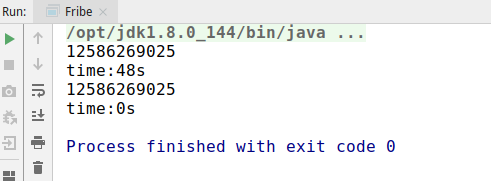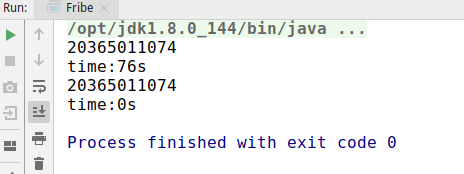可以看到，在计算第50项的时候，第一种递归方式花费了48秒的时间，而第二种不到一秒，虽然这种方式不太科学，但也看出来了两者巨大的差距，并且随着计算的值越大，时间的差异越明显。由此可见，时间复杂度是决定一个算法好坏的重要指标。
如何衡量一个算法的好坏
正确性、可读性、健壮性。 算法必须要保证正确，不正确的算法是没有必要衡量其好坏的；算法也要保证良好的可读性，能够让阅读者明白内在实现与逻辑；健壮性为对不合理输入的反应能力和处理能力，比如非法输入，要有相应的处理，而不应该程序奔溃等。这些都是一个良好的算法必备的条件。时间复杂度 时间复杂度也是一个衡量算法优劣的重要条件，不同的算法的执行时间可能会存在很大的差别。空间复杂度 空间复杂度表示一个算法执行过程中，需要的空间(内存)数量，也是衡量一个算法的重要指标，尤其是在嵌入式等程序中的算法，内存是非常宝贵的，有时候宁愿提高时间复杂度，也要保证不占用太多的空间。
如何计算时间复杂度
第一种：事后统计法
上面我们使用了一种计算执行前后时间差的方式，直观的来看一个算法的复杂度，比较不同算法对同一组输入的执行时间，这种方法也叫作"事后统计法"，但是这种方法也存在一些问题，主要问题有：
执行时间严重依赖于硬件已经运行时各种不确定的环境因素。 比如两个算法在不同的硬件机器上进行测试，硬件不同，运行时间也会存在差异，即使就在一台机器上执行，也会存在运行时机器的CPU、内存使用情况不同等因素。必须要编写相应的测试代码。测试数据的选择难以保证公正性。 比如有两个算法，一个在数据量小的时候占优，一个在大数据量的时候运行较快，这样便难以选择一个公正的测试数据。
第二种：估算代码指令执行次数
那么我们可以使用代码的每个指令的执行次数，可以简单估算代码的执行次数，一般情况下，执行次数少的肯定要比执行次数多的花的时间更少。看如下的示例：
    public static void test1(int n) {
if (n > 10) {
System.out.println("n > 10");
} else if (n > 5) {
System.out.println("n > 5");
} else {
System.out.println("n <= 5");
}

for (int i = 0; i < 4; i++) {
System.out.println("test");
}
}

上面这个方法，我们计算它的执行次数。
最上面的if…else if…else这个判断，判断会执行一次、判断成立的代码会执行一次。下面的for循环，i=0这句赋值会执行一次，i<4这个判断条件会执行4次，i++也会执行4次，循环体(输出语句)也会执行4次。因此，整个方法的执行次数为：1+1+1+4+4+4 = 15次。
    public static void test2(int n) {
for (int i = 0; i < n; i++) {
System.out.println("test");
}
}

上面这个方法，我们计算它的执行次数。
在for循环中，i=0这句赋值会执行一次，i < n执行n次，i++执行n次，循环体执行n次。因此，整个方法的执行次数为：1+n+n+n = 3n+1 次
    public static void test3(int n) {
for (int i = 0; i < n; i++) {
for (int j = 0; j < n; j++) {
System.out.println("test");
}
}
}

上面这个方法，我们计算它的执行次数。
在外层for循环中，i=0这句赋值会执行一次，i < n执行n次，i++执行n次，循环体执行n次。在内层循环中，j=0这句赋值会执行一次，j < n执行n次，j++执行n次，循环体执行n次。因此，整个方法的执行次数为 1+n+n+n*(1+n+n+n)=3n2+3n+1 次
    public static void test4(int n) {
for (int i = 0; i < n; i++) {
for (int j = 0; j < 15; j++) {
System.out.println("test");
}
}
}


上面这个方法，我们计算它的执行次数。
在外层for循环中，i=0这句赋值会执行一次，i < n执行n次，i++执行n次，循环体执行n次。在内层循环中，j=0这句赋值会执行一次，j < 15执行15次，j++执行15次，循环体执行15次。因此，整个方法的执行次数为 1+n+n+n*(1+15+15+15)=48n+1 次
    public static void test5(int n) {
while ((n = n / 2) > 0) {
System.out.println("test");
}
}

上面这个方法，我们计算它的执行次数。
在while循环中，每次对n取一半，相当于对n取以二为底的对数，因此n = n / 2 会执行log2(n)次，判断条件也会执行log2(n)次。在循环体中，这个输出语句也会执行log2(n)次。因此，整个方法的执行次数为 log2(n) + log2(n) + log2(n) = 3log2(n)次
    public static void test6(int n) {
while ((n = n / 5) > 0) {
System.out.println("test");
}
}

上面这个方法，我们计算它的执行次数。
在while循环中，每次对n取五分之一，相当于对n取以五为底的对数，因此n = n / 5 会执行log5(n)次，判断条件也会执行log5(n)次。在循环体中，这个输出语句也会执行log5(n)次。因此，整个方法的执行次数为 log5(n) + log5(n) + log5(n) = 3log5(n)次
    public static void test7(int n) {
for (int i = 1; i < n; i = i * 2) {
for (int j = 0; j < n; j++) {
System.out.println("test");
}
}
}

上面这个方法，我们计算它的执行次数。
在外层for循环中，i= 1执行一遍，每次i翻倍，执行次数为log2(n)，因此i < n会执行log2(n)次，i=i*2会执行log2(n)次，循环体执行log2(n)。在内层for循环中，j=0执行一次，j < n执行n次，j++执行n次，内层循环条件执行n次。因此，整个方法的执行次数为 1+ log2(n) + log2(n) + log2(n)*(1+n+n+n) = 3nlog2(n) + 3log2(n)+1次
    public static void test8(int n) {
int a = 10;
int b = 20;
int c = a + b;
int[] array = new int[n];
for (int i = 0; i < array.length; i++) {
System.out.println(array[i] + c);
}
}

上面这个方法，我们计算它的执行次数。
a=10执行一次，b=20执行一次，c=a+b执行一次，初始化数组执行一次。在for循环中，i=0执行一次，i < 数组长度执行n次，i++执行n次，内层循环条件执行n次。因此，整个方法的执行次数为 1+1+1+1+1+n+n+n =3n +5次。
使用这种方法我们发现计算会特别麻烦，而且不同的时间复杂度表达书也比较复杂，我们也不好比较两个时间复杂度的具体优劣，因此为了更简单、更好的比较不同算法的时间复杂度优劣，提出了一种新的时间 复杂度表示法—大O表示法。
大O表示法

大O表示法：算法的时间复杂度通常用大O符号表述，定义为T[n] = O(f(n))。称函数T(n)以f(n)为界或者称T(n)受限于f(n)。 如果一个问题的规模是n，解这一问题的某一算法所需要的时间为T(n)。T(n)称为这一算法的“时间复杂度”。当输入量n逐渐加大时，时间复杂度的极限情形称为算法的“渐近时间复杂度”。

大O表示法，用来描述复杂度，它表示的是数据规模n对应的复杂度，大O表示法有以下的一些特性：
忽略表达式常数、系数、低阶项。 忽略常数，常数直接为1，比如上面第一个方法的复杂度为15，因此直接取1，其时间复杂度使用大O表示为O(1)。 忽略系数，忽略表达式的系数，比如第二个方法的时间复杂度为3n+1，忽略系数和常数，其时间复杂度为O(n)。 忽略低阶项，比如第三个方法的时间复杂度为3n2+3n+1,忽略低阶项3n，忽略常数1，忽略系数3，则其时间复杂度为O(n2)。对数阶一般忽略底数 对于对数直接的转换，一个对数都可以乘以一个常数项成为一个没有底数的对数，比如 log2n = log29 * log9n，因此可以省略底数，比如上面第五个方法的时间复杂度为log2(n),可以忽略底数2，则其时间负责度为logn。大O表示法仅仅是一种粗略的分析模型，是一种估算，能帮助我们短时间内估算一个算法的时间复杂度。
常见的复杂度
执行次数复杂度非正式术语12O(1)常数阶2n+3O(n)线性阶4n2+zn+2O(n2)平方阶4log2n+21O(logn)对数阶3n+2log3n+15O(nlogn)nlogn阶4n3+3n2+22n+11O(n3)立方阶2nO(2n)指数阶
复杂度的大小关系 O(1) < O(logn) < O(n) < O(nlogn) < O(n2) < O(n3) < O(2n) < O(n!) < O(nn)。
因此上面的十个方法的复杂度如下：
方法名称复杂度大O表式test115O(1)test23n+1O(n)test33n2+3n+1O(n2)test448n+1O(n)test53log2(n)O(logn)test63log5(n)O(logn)test73nlog2(n) + 3log2(n) + 1O(nlogn)test83n+5O(n)
直观对比复杂的的大小
直接看表达式，还是很难判断一些复杂度的大小关系，我们可以借助可视化的一些工具来查看比如https://zh.numberempire.com/graphingcalculator.php，通过该网站我们看到在n变化的情况下，不同表达式的变换情况。
递归斐波拉契数列计算方法的时间复杂度分析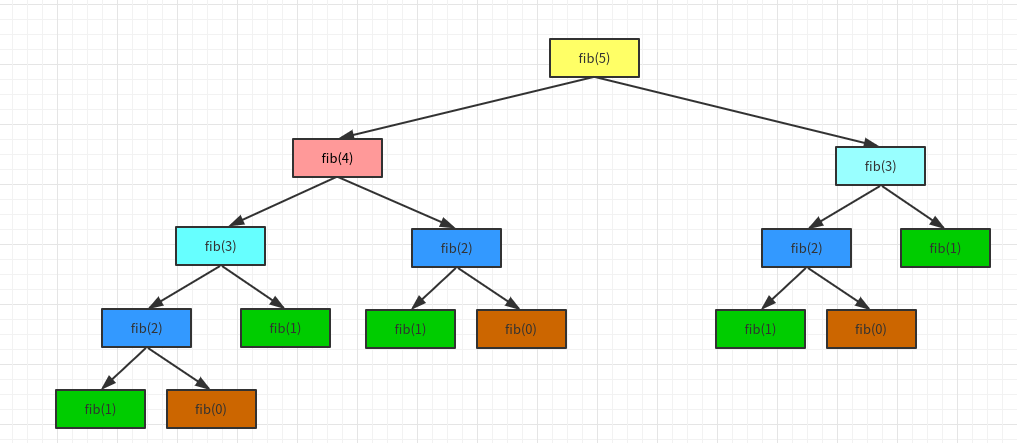第一层计算5，只需要计算1次；第二层计算3和4，2次；计算第3层，4次；计算第4层，8次。所以总共计算1+2+4+8 =15= 25-1 = 1/2 * 22 -1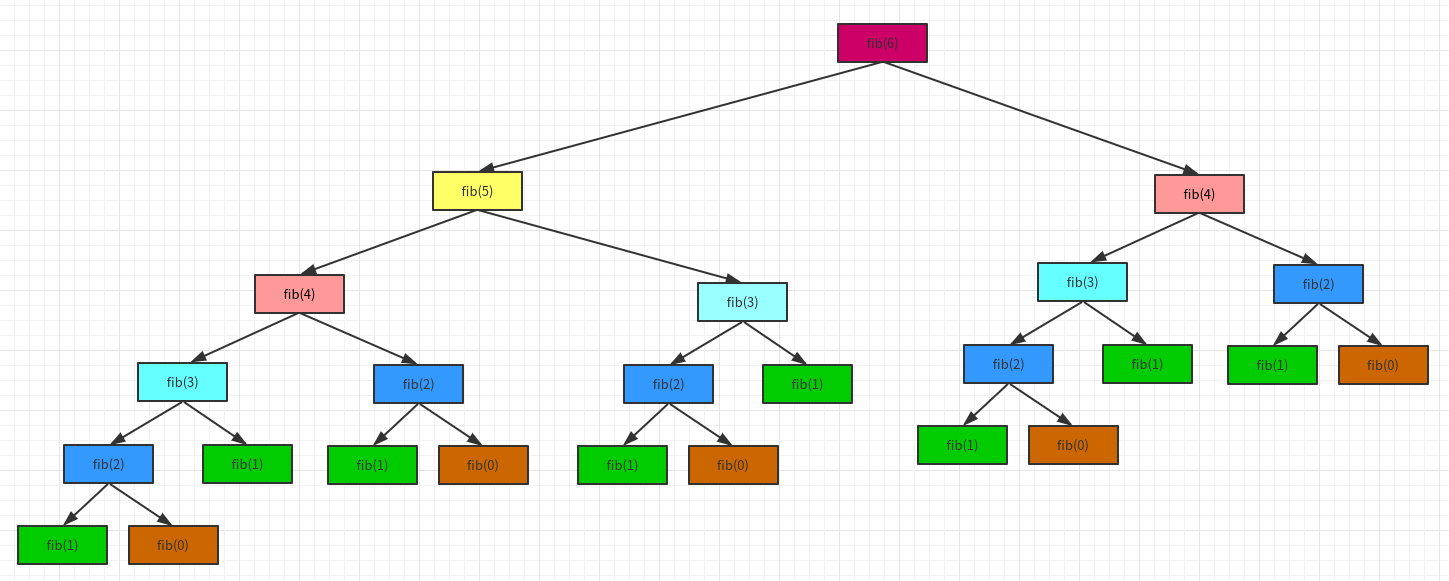第一层计算6，只需要计算1次；第二层计算5和4，2次；计算第3层，4次；计算第4层，8次；第5层，计算10次。所以总共计算1+2+4+8+10 =25 = 25 - 7 = 1/2 * 26 - 7。 所以计算第n项，它的时间复杂度为O(2^n)。 所以最开始的两个算法，第一个的算法复杂度为O(2n),一个为O(n)。 他们的差别有多大？
如果有一台1GHz的普通计算机，运算速度109次每秒（n为64）O(n)大约耗时6.4 ∗ 10-8秒O(2n)大约耗时584.94年有时候算法之间的差距，往往比硬件方面的差距还要大
算法的优化方向
用尽量少的存储空间，即空间复杂度低。用尽量少的执行步骤，即时间复杂度低。一定情况下，时间复杂度和空间复杂度可以互换。
关于复杂度的更多概念
最好、最坏复杂度均摊复杂度复杂度震荡平均复杂度…
总结展开全文java
• 算法复杂度分为时间复杂度和空间复杂度。 时间复杂度用于度量算法执行的时间长短；而空间复杂度则是用于度量算法所需存储空间的大小。 目录 时间复杂度 1.时间频度 2.计算方法 3.分类 空间复杂度 算法时间...
文章地址：http://lzw.me/a/algorithm-complexity.html
算法复杂度分为时间复杂度和空间复杂度。 时间复杂度用于度量算法执行的时间长短；而空间复杂度则是用于度量算法所需存储空间的大小。
目录
时间复杂度
1.时间频度
2.计算方法
3.分类
空间复杂度
算法的时间复杂度（计算实例）
算法复杂度的渐近表示法
一  大O记号
二  Ω记号
三  Θ记号
四  小o记号
五  例子
常见排序算法时空复杂度

时间复杂度
1.时间频度
一个算法执行所耗费的时间，从理论上是不能算出来的，必须上机运行测试才能知道。但我们不可能也没有必要对每个算法都上机测试，只需知道哪个算法花费的时间多，哪个算法花费的时间少就可以了。并且一个算法花费的时间与算法中语句的执行次数成正比例，哪个算法中语句执行次数多，它花费时间就多。一个算法中的语句执行次数称为语句频度或时间频度。记为T(n)。
2.计算方法
1. 一般情况下，算法的基本操作重复执行的次数是模块n的某一个函数f（n），因此，算法的时间复杂度记做：T（n）=O（f（n）） 　　分析：随着模块n的增大，算法执行的时间的增长率和f（n）的增长率成正比，所以f（n）越小，算法的时间复杂度越低，算法的效率越高。
2. 在计算时间复杂度的时候，先找出算法的基本操作，然后根据相应的各语句确定它的执行次数，再找出T（n）的同数量级（它的同数量级有以下：1，Log2n ，n ，nLog2n ，n的平方，n的三次方，2的n次方，n！），找出后，f（n）=该数量级，若T(n)/f(n)求极限可得到一常数c，则时间复杂度T（n）=O（f（n））
例：算法：

for（i=1;i<=n;++i） 　　{ 　　for(j=1;j<=n;++j) 　　{ 　　c[ i ][ j ]=0; //该步骤属于基本操作执行次数：n的平方 次 　　for(k=1;k<=n;++k) 　　c[ i ][ j ]+=a[ i ][ k ]*b[ k ][ j ]; //该步骤属于基本操作 执行次数：n的三次方 次 　　} 　　}

则有 T（n）= n的平方+n的三次方，根据上面括号里的同数量级，我们可以确定 n的三次方 为T（n）的同数量级 　　则有f（n）= n的三次方，然后根据T（n）/f（n）求极限可得到常数c 　　则该算法的 时间复杂度：T（n）=O（n的三次方）
3.分类
按数量级递增排列，常见的时间复杂度有： 　　常数阶O(1),对数阶O(log2n),线性阶O(n), 　　线性对数阶O(nlog2n),平方阶O(n2)，立方阶O(n3),…， 　　k次方阶O(nk), 指数阶O(2n) 。随着问题规模n的不断增大，上述时间复杂度不断增大，算法的执行效率越低。
空间复杂度
与时间复杂度类似，空间复杂度是指算法在计算机内执行时所需存储空间的度量。记作: 　　S(n)=O(f(n)) 　　我们一般所讨论的是除正常占用内存开销外的辅助存储单元规模。
算法的时间复杂度（计算实例）
算法的时间复杂度 定义：如果一个问题的规模是n，解这一问题的某一算法所需要的时间为T(n)，它是n的某一函数 T(n)称为这一算法的“时间复杂性”。
当输入量n逐渐加大时，时间复杂性的极限情形称为算法的“渐近时间复杂性”。
我们常用大O表示法表示时间复杂性，注意它是某一个算法的时间复杂性。大O表示只是说有上界，由定义如果f(n)=O(n)，那显然成立f(n)=O(n^2)，它给你一个上界，但并不是上确界，但人们在表示的时候一般都习惯表示前者。
此外，一个问题本身也有它的复杂性，如果某个算法的复杂性到达了这个问题复杂性的下界，那就称这样的算法是最佳算法。
“大O记法”：在这种描述中使用的基本参数是 n，即问题实例的规模，把复杂性或运行时间表达为n的函数。这里的“O”表示量级 (order)，比如说“二分检索是 O(logn)的”,也就是说它需要“通过logn量级的步骤去检索一个规模为n的数组”记法 O ( f(n) )表示当 n增大时，运行时间至多将以正比于 f(n)的速度增长。
这种渐进估计对算法的理论分析和大致比较是非常有价值的，但在实践中细节也可能造成差异。例如，一个低附加代价的O(n2)算法在n较小的情况下可能比一个高附加代价的 O(nlogn)算法运行得更快。当然，随着n足够大以后，具有较慢上升函数的算法必然工作得更快。
O(1)
Temp=i;i=j;j=temp;
以上三条单个语句的频度均为1，该程序段的执行时间是一个与问题规模n无关的常数。算法的时间复杂度为常数阶，记作T(n)=O(1)。如果算法的执行时间不随着问题规模n的增加而增长，即使算法中有上千条语句，其执行时间也不过是一个较大的常数。此类算法的时间复杂度是O(1)。
O(n^2)
2.1. 交换i和j的内容

sum=0； （一次） for(i=1;i<=n;i++) （n次） for(j=1;j<=n;j++) （n^2次） sum++； （n^2次）

解：T(n)=2n^2+n+1 =O(n^2)
2.2.

for (i=1;i<n;i++) { y=y+1; ①  for (j=0;j<=(2*n);j++)  x++; ②  }

解：语句1的频度是n-1 语句2的频度是(n-1)*(2n+1)=2n^2-n-1 f(n)=2n^2-n-1+(n-1)=2n^2-2 该程序的时间复杂度T(n)=O(n^2).
O(n)

2.3. a=0; b=1; ① for (i=1;i<=n;i++) ② {  s=a+b;　　　　③ b=a;　　　　　④  a=s;　　　　　⑤ }

解：语句1的频度：2,  语句2的频度： n,  语句3的频度： n-1,  语句4的频度：n-1,  语句5的频度：n-1,  T(n)=2+n+3(n-1)=4n-1=O(n).
O(log2n )
2.4.

i=1; ① while (i<=n) i=i*2; ②

解： 语句1的频度是1,  设语句2的频度是f(n), 则：2^f(n)<=n;f(n)<=log2n  取最大值f(n)= log2n, T(n)=O(log2n )
O(n^3)
2.5.

for(i=0;i<n;i++) {  for(j=0;j<i;j++)  { for(k=0;k<j;k++) x=x+2;  } }

解：当i=m, j=k的时候,内层循环的次数为k当i=m时, j 可以取 0,1,…,m-1 , 所以这里最内循环共进行了0+1+…+m-1=(m-1)m/2次所以,i从0取到n, 则循环共进行了: 0+(1-1)*1/2+…+(n-1)n/2=n(n+1)(n-1)/6所以时间复杂度为O(n^3).
我们还应该区分算法的最坏情况的行为和期望行为。如快速排序的最坏情况运行时间是 O(n^2)，但期望时间是 O(nlogn)。通过每次都仔细地选择基准值，我们有可能把平方情况 (即O(n^2)情况)的概率减小到几乎等于 0。在实际中，精心实现的快速排序一般都能以 (O(nlogn)时间运行。
下面是一些常用的记法：
访问数组中的元素是常数时间操作，或说O(1)操作。一个算法如 果能在每个步骤去掉一半数据元素，如二分检索，通常它就取 O(logn)时间。用strcmp比较两个具有n个字符的串需要O(n)时间。常规的矩阵乘算法是O(n^3)，因为算出每个元素都需要将n对 元素相乘并加到一起，所有元素的个数是n^2。
指数时间算法通常来源于需要求出所有可能结果。例如，n个元 素的集合共有2n个子集,所以要求出所有子集的算法将是O(2n)的。指数算法一般说来是太复杂了，除非n的值非常小，因为，在 这个问题中增加一个元素就导致运行时间加倍。不幸的是，确实有许多问题 (如著名的“巡回售货员问题” )，到目前为止找到的算法都是指数的。如果我们真的遇到这种情况，通常应该用寻找近似最佳结果的算法替代之。
算法复杂度的渐近表示法
一个算法的时间复杂度，指算法运行的时间。
假设数据输入规模是n，算法的复杂度可以表示为f(n)的函数
一  大O记号
假设f(n)和g(n)的定义域是非负整数，存在两个正整数c和n0，使得n>n0的时候，f(n)≤c*g(n),则f(n)=O(g(n))。可见O(g(n))可以表示算法运行时间的上界。O(g(n))表示的函数集合的函数是阶数不超过g(n)的函数。
例如：f(n)=2*n+2=O(n)
证明：当n>3的时候，2*n +2<3n，所以可选n0=3,c=3，则n>n0的时候，f(n)<c*(n)，所以f(n)=O(n)。
现在再证明f(n)=2*n+2=O(n^2)
证明：当n>2的时候，2*n+2<2*n^2，所以可选n0=2,c=2,则n>n0的时候，f(n)<c*(n^2)，所以f(n)=O(n^2)。
同理可证f(n)=O(n^a)，a>1
二  Ω记号
Ω记号与大O记号相反，他可以表示算法运行时间的下界。Ω（g（n））表示的函数集合的函数是所有阶数超过g(n)的函数。
例如：f(n)=2*n^2+3*n+2=Ω(n^2)
证明：当n>4的时候，2*n^2+3*n+2>n^2，所以可选n0=4,c=1,则n>n0的时候，f(n)>c*(n^2)，所以f(n)=Ω(n^2)。
同理可证f(n)=Ω(n),f(n)=Ω(1)
三  Θ记号
Θ记号介于大O记号和Ω记号之间。他表示，存在正常数c1,c2,n0，当n>n0的时候，c1*g(n)≤f(n)≤c2*g(n)，则f(n)=Θ(g(n))。他表示所有阶数与g(n)相同的函数集合。
四  小o记号
f(n)=o(g(n))当且仅当f(n)=O(g(n))且f(n)≠Ω(g(n))。也就是说小o记号可以表示时间复杂度的上界，但是一定不等于下界。
五  例子
假设f(n)=2n^2+3n+5，
则f(n)=O(n^2)或者f(n) = O(n^3)或者f(n)=O(n^4)或者……
f(n)=Ω(n^2)或者f(n)=Ω(n)或者f(n)=Ω(1)
f(n)=Θ(n^2)
f(n) = o(n^3)或者f(n)=o(n^4)或者f(n)=o(n^5)或者……
注：n^2表示n的平方，以此类推。
常见排序算法时空复杂度

排序法 最差时间分析平均时间复杂度稳定度空间复杂度冒泡排序O(n2)O(n2)稳定O(1)快速排序O(n2)O(n*log2n)不稳定O(log2n)~O(n)选择排序O(n2)O(n2)稳定O(1)二叉树排序O(n2)O(n*log2n)不一顶O(n) 插入排序 O(n2)O(n2)稳定O(1)堆排序O(n*log2n)O(n*log2n)不稳定O(1)希尔排序OO不稳定O(1)
展开全文计算机领域 复杂度 空间复杂度
• ## 算法时间复杂度计算方式

万次阅读 多人点赞 2019-04-09 14:53:00
算法的正确性评估不在本文范围之内，本文主要讨论从算法时间复杂度特性去评估算法的优劣。】 如何衡量一个算法的好坏呢？ 显然，选用的算法应该是正确的（算法的正确性不在此论述）。除此之外，通常有三个方面的...
• 一、算法时间复杂度定义 在进行算法分析时候,语句总的执行次数T(n)是关于问题规模n的函数,进而分型T(n)随着n的变化情况并确定T(n)的数量级.算法的时间复杂度,也就是算法的时间度量记作:T(n)=O(f(n)).它表示随着...
•递归
• 算法设计与复杂度分析 算法复杂性分析 算法复杂性是算法运行所需要的计算机资源的量 需要时间资源的量称为时间复杂性,需要的空间资源的 量称为空间复杂性这个量应该只依赖于算法要解的 题的规模算法的输入和算法本身...
• 复杂度分析简介 常数项不计入复杂度 ...当数据规模小的时候，...但是要注意两部分的n是否相同，n不同时间复杂度由两部分组成： 数据规模 复杂度：O(n) 数据规模每上升10倍，时间增加十倍 ...
• O(1)<O(log2n)<O(n)<O(nlog2n)<O(n^2)<O(n^3)<O(2^n)<O(n!)<O(n^n)数据结构
• 为了达到此目的，必须熟悉算法时间复杂度与问题规模之间的关系。 常见的对应关系如下图所示。即若给出了某种问题规模，那么只能设计出对应的时间复杂度的算法，才能不超限。 【参考文献】 ...
•数据结构
• ## C++算法篇 算法时间复杂度图解

千次阅读 多人点赞 2019-12-22 16:00:09
究竟什么是时间复杂度呢？让我们来想象一个场景：某一天，小灰和大黄同时加入了一个公司...... 一天过后，小灰和大黄各自交付了代码，两端代码实现的功能都差不多。大黄的代码运行一次要花100毫秒，内存占用5MB...
• (1) 复杂度 为了描述一个算法的优劣，我们引入算法时间复杂度和空间复杂度的概念。 时间复杂度：一个算法主要运算的次数，用大 O 表示。通常表示时间复杂度时，我们只保留数量级最大的 项，并忽略该项的系数。 例如...
• ## 详细概述算法时间复杂度(C语言)

千次阅读 多人点赞 2019-12-01 18:32:43
其实，在学它之前，自己也会这样想，现在电脑中CPU运行速度这么快且相关的性能也显著提高，为什么还要去学习怎么去提高算法效率，怎么去计算或者表示算法时间复杂度呢？当然，上述的话还不无道理，然而，当你能够去...数据结构 C语言
• 2. 如果是递归算法，且只进行一次递归调用，有以一种方法是先求出深度depth，求出每一次执行的时间复杂度T ，总的时间复杂度就是depth * T（和用下面的方法原理是一样的。。） 如果递归比较复杂，那么套用递归算法...
• 算法时间复杂度定义 在进行算法分析时，语句总的执行次数T(n)是关于问题规模n的函数，进而分析T(n)随n的变化情况并确定T(n)的数量级。算法的时间复杂度，也就是算法的时间量度，记作：T(n)=O(f(n))。f(n)是问题规模n...
• 都别说了，听我的，我看了很多资料和大佬的blog，最后确认王道的那个视频错了 最好时间复杂度o（1） 最差时间复杂度o（mn）...